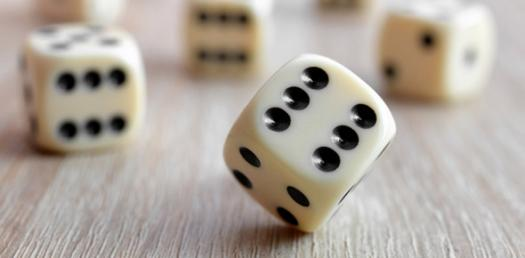# Probability Of Single And Muiltiple Events

Approved & Edited by ProProfs Editorial Team
At ProProfs Quizzes, our dedicated in-house team of experts takes pride in their work. With a sharp eye for detail, they meticulously review each quiz. This ensures that every quiz, taken by over 100 million users, meets our standards of accuracy, clarity, and engagement.
| Written by Pedgerton
P
Pedgerton
Community Contributor
Quizzes Created: 1 | Total Attempts: 340
Questions: 9 | Attempts: 340SettingsA test of probability knowledge for Y9 students.

• 1.

### A probability of 1 means an outcome is:

• A.

Not likely to happen

• B.

Probably going to happen

• C.

Absolutely certain

• D.

Possibly going to happen

C. Absolutely certain
Explanation
A probability of 1 means that the outcome is absolutely certain. This means that there is a 100% chance that the event will occur. There is no doubt or uncertainty involved, and the outcome is guaranteed to happen.

Rate this question:

• 2.

### A card is taken at random from a pack of 52 playing cards; what is the probability that it is a Red 7?

• A.

1/13

• B.

1/52

• C.

1/26

C. 1/26
Explanation
The probability of drawing a red 7 from a pack of 52 playing cards can be calculated by dividing the number of red 7 cards (2) by the total number of cards in the pack (52). Therefore, the probability is 2/52, which simplifies to 1/26.

Rate this question:

• 3.

### If you roll a fair dice 120 times how many times would you expect to get a 4?

• A.

50

• B.

60

• C.

20

C. 20
Explanation
If you roll a fair dice 120 times, you would expect to get a 4 approximately 1/6th of the time since there are 6 possible outcomes (numbers 1 to 6) and each has an equal chance of occurring. Therefore, 1/6th of 120 is 20, so you would expect to get a 4 20 times.

Rate this question:

• 4.

### The faces of a regular tetrahedron are numbered 1 to 4. When rolled it lands face down on one of these numbers. What is the probability that this number is not 2?

• A.

1/4

• B.

1/2

• C.

3/4

• D.

1

C. 3/4
Explanation
The regular tetrahedron has four faces numbered 1 to 4. Since each face has an equal chance of landing face down when rolled, the total number of possible outcomes is 4. Out of these four outcomes, only one outcome corresponds to landing on face 2. Therefore, the probability of not landing on face 2 is 3 out of 4, which can be simplified to 3/4.

Rate this question:

• 5.

### Using the same dice as in question 5, what is the probability that the score is at least 8?

• A.

1/3

• B.

7/36

• C.

1/4

• D.

5/12

D. 5/12
Explanation
The probability of getting a score of at least 8 can be calculated by finding the sum of probabilities of getting a score of 8, 9, 10, 11, or 12. From question 5, we know that the probabilities of getting each of these scores are 5/36, 4/36, 3/36, 2/36, and 1/36 respectively. Adding these probabilities together gives us 5/36 + 4/36 + 3/36 + 2/36 + 1/36 = 15/36 = 5/12. Therefore, the probability of getting a score of at least 8 is 5/12.

Rate this question:

• 6.

### And again, with the two dice, what is the probability of a double being scored?

• A.

1/3

• B.

1/5

• C.

1/6

• D.

1/4

C. 1/6
Explanation
The probability of a double being scored with two dice can be determined by calculating the number of favorable outcomes (where both dice show the same number) divided by the total number of possible outcomes. Since there are 6 possible outcomes for each die (1, 2, 3, 4, 5, 6), there are 6 favorable outcomes (1-1, 2-2, 3-3, 4-4, 5-5, 6-6) out of a total of 36 possible outcomes (6 x 6). Therefore, the probability of a double being scored is 1/6.

Rate this question:

• 7.

### And finally, with the two dice, what is the probability of a score of 10 or more given that one of the dice shows a 6?

• A.

1/2

• B.

5/36

• C.

5/11

• D.

3/11

C. 5/11
Explanation
Given that one of the dice shows a 6, there are 11 possible outcomes since the other dice can show any number from 1 to 6. Out of these 11 outcomes, there are 5 ways to get a score of 10 or more (6+4, 6+5, 6+6, 4+6, 5+6). Therefore, the probability of a score of 10 or more given that one of the dice shows a 6 is 5/11.

Rate this question:

• 8.

### A coin is biased so that the probability of a Head is 3/5. What is the probability of getting two heads with two tosses of the coin?

• A.

9/25

• B.

6/25

• C.

6/10

• D.

4/25

A. 9/25
Explanation
The probability of getting a head on one toss of the biased coin is 3/5. Since the two tosses are independent events, the probability of getting two heads in a row is the product of the individual probabilities. Therefore, the probability of getting two heads with two tosses of the coin is (3/5) * (3/5) = 9/25.

Rate this question:

• 9.

### Finally, with the same biased coin, with three tosses, what is the probility of at least one tail?

• A.

3/5

• B.

27/125

• C.

98/125

• D.

17/125Back to top